# WEEKDAY, DAY and other functions to calculate days in Excel

In this tutorial, you will find lots of formula examples demonstrating the use of WEEKDAY, DAY and DAYS functions in Excel. See how to return a day of week from date, get the number of days in the year, find how many days are between two dates and more.

There are a variety of functions to work with days of year and days of the week in Excel, including WEEKDAY, DAY, DAYS, DATE and more. These functions can be used to determine workdays and weekends, calculate the number of days between two dates, count the number of days remaining in the year, return day of the week from date, and much more.

Weekday Excel functions are particularly useful for planning and scheduling, for example to determine the timeframe of a project and automatically remove weekend days from the total. So, let's run through the functions one-at-a-time and see how they can help you cope with various tasks in Excel.

## Working with days of week (Excel WEEKDAY function)

Microsoft Excel provides a special WEEKDAY function to return the day of the week corresponding to a given date.

The result returned by an Excel WEEKDAY formula is an integer, ranging from 1 (Sunday) to 7 (Saturday) by default. If your formula's logic requires a different enumeration, you can start counting with any day of week, as you will see in a moment.

The syntax of the Excel WEEKDAY function is as follows:

WEEKDAY(serial_number,[return_type])
• Serial_number - a serial number that represents the date, or a reference to a cell with a date or serial number.

If the term "serial number" does not make much sense to you, let me remind you that Excel stores all dates as serial numbers beginning with January 1, 1900, which is stored as number 1. And it is these numbers that the WEEKDAY function operates on. If you want to learn more about how Excel stores dates and times, you may find the following article useful: Excel date format.

• Return_type (optional) - determines what day of the week to use as the first day in calculations.

In your Excel WEEKDAY formulas, you can specify any of the following values for the return_type argument:

 Return_type Number returned 1 or omitted From 1 (Sunday) to 7 (Saturday) 2 From 1 (Monday) to 7 (Sunday) 3 From 0 (Monday) to 6 (Sunday) 11 From 1 (Monday) to 7 (Sunday) 12 From 1 (Tuesday) to 7 (Monday) 13 From 1 (Wednesday) to 7 (Tuesday) 14 From 1 (Thursday) to 7 (Wednesday) 15 From 1 (Friday) to 7 (Thursday) 16 From 1 (Saturday) to 7 (Friday) 17 From 1 (Sunday) to 7 (Saturday)
Note. Though the WEEKDAY function is available in all Excel versions, from Excel 2013 to 2000, the return_type values 11 through 17 were introduced in Excel 2010 only, therefore they cannot be used in earlier versions.

And now, let's look at some examples of using the WEEKDAY function in Excel. All of the below formulas return the day of the week corresponding to March 19, 2015. For the sake of clarity, this date is stored as number 42082 in Excel.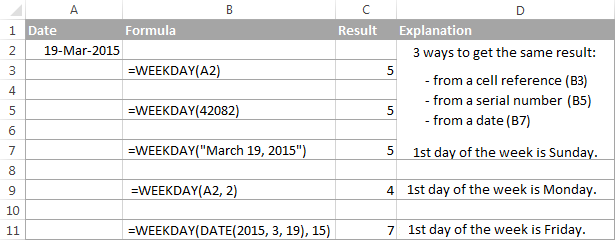At first sight, it may seems that the numbers returned by the Excel WEEKDAY function have very little practical sense. But let's look at it from a different angle and discuss some formulas that solve real-life tasks.

#### Example 1. Excel WEEKDAY formula to find workdays and weekends

If you have a long date column in your worksheet, you may want to know which dates are working days and which are weekends.

An easiest way is to have a day name displayed in a cell, for example as "Friday, March 20, 2015" or just "Friday". And you can do this in no time by simply changing the date format. However, this is not always an ideal approach, firstly, because a short date format is often required, and secondly, because you may need to filter only weekends or only workdays in your Excel list.

One of possible solutions is embedding the WEEKDAY function into the logical test of the IF function:

`=IF(WEEKDAY(A2,2)<6, "Workday", "Weekend")`

In this Weekday formula, we set the `return_type ` argument to 2, which corresponds to the week beginning with Monday (day 1). So, if the day of the week in cell A2 is less than 6 (Monday through Friday), the formula returns "Workday", otherwise - "Weekend".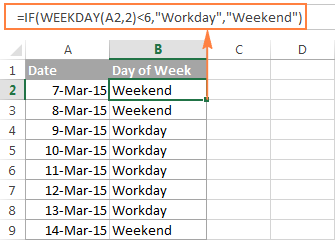To filter weekdays or weekend days, you apply Excel's filter to your table (Data tab > Filter) and select either "Workday" or "Weekend".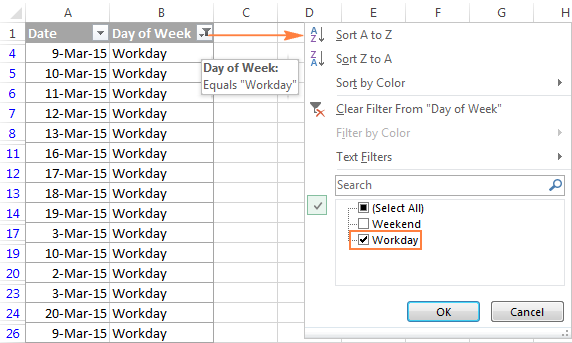If your organization, or maybe some regional office of your company, works on a different schedule where the days of rest are other than Saturday and Sunday, you can easily adjust this WEEKDAY formula to your needs by specifying a different `return_type `.

For example, to treat Saturday and Monday as weekends, you set return_type to 12, since you want the "Tuesday (1) to Monday (7)" week type:

`=If(WEEKDAY(A2, 12)<6, "Weekday", "Weekend")`

#### Example 2. How to highlight workdays and weekends in Excel

The Excel WEEKDAY formula discussed in the previous example has no problem with finding working days and weekends, no matter which days of the week are days off. However, you can improve the visual presentation of the results by shading workings days and weekend days in different colors.

For this, you can create Excel conditional formatting rules with the following WEEKDAY formulas to highlight weekends or workdays, or both:

Highlight weekends (Saturday and Sunday): `=WEEKDAY(\$A2,2)<6`

Highlight workdays (Monday - Friday): `=WEEKDAY(\$A2,2)>5`

The results look much better now, don't they?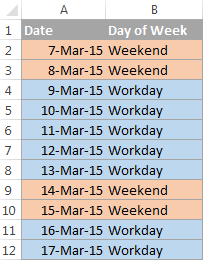#### Example 3. How to get a day of the week from date in Excel

If you have a list of dates in Excel and you aim to find a day of week for each date, you do not actually need any special formulas :) The point is that Excel already knows what day of week a given date is and all you need to do is get it to display that information.

For example, if your dates are in column A, you can put a simple =A2 formula in cell B2 and then copy it down to other cells. After that, you select the entire column B and set the custom date format to it, such as:

• ddd - to display an abbreviated day name, e.g. Sun.
• dddd - to display full day names, e.g. Sunday.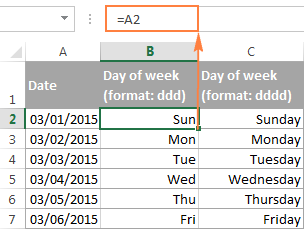Please note that the results, which may look like usual text entries to you, are in fact fully functional Excel dates that you can use in other calculations. You can tell these are dates by their right alignment in a cell as opposed to left-aligned text values.

#### Example 4. Return day of week as a text value

An alternative way to determine a day of the week by date is using the Excel TEXT function. In your TEXT formulas, you can specify the same formats as in the above example - "ddd" to return a short name and "dddd" to return full names of the days of the week:

`=TEXT(A1, "ddd")`

`=TEXT(A1,"dddd")`

Where A1 is a cell with the original date.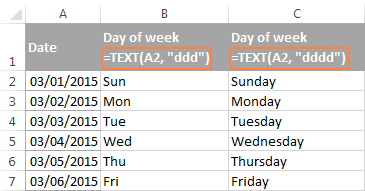When using this method, please remember that the TEXT function in Excel always returns text strings regardless of the source cell's format. So, the day of week names you see in the above screenshot are text entries, and not dates. And this is what makes them different from the results of the previous example, which are still fully functional Excel dates.

#### Example 5. How to return a custom day name in Excel

Yet another way to find the day of the week from date in Excel is using the WEEKDAY function in liaison with CHOOSE. An advantage of this formula is that it lets you return the day names in any format of your choosing.

For example, if you want to display days of week as the first 2 letters, enter them as `value` arguments in the CHOOSE function:

`=CHOOSE(WEEKDAY(A2),"Su","Mo","Tu","We","Th","Fr","Sa")`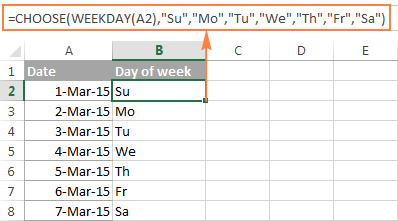In this formula, the Excel WEEKDAY function gets the day of week as a serial number. And the CHOOSE function uses that number as `index_num` (the 1st argument) that indicates which value from the list of `value` arguments to return.

## How to get the day of month from date (DAY function)

Microsoft Excel provides a special DAY function to retrieve the day of the month from a date:

DAY(serial_number)

The day is returned as a serial number ranging from 1 to 31. For example, in you have a list of dates in column A and you want to extract the days into column B, you put the following formula in cell B2 and then copy it down to other cells:

`=DAY(A2)`

Note. The Excel DAY function correctly handles dates in different Date formats, though the problems may occur if dates are entered as text. Please see How to distinguish normal Excel dates from text dates for full details.

An alternative way to extract a day of the month from a date is using the TEXT function, for example:

`=TEXT(A2, "d")` - to display day numbers without leading zeros, or

`=TEXT(A2, "dd")` - to display day numbers with leading zeros.

Note. The Excel TEXT function always returns text strings, not numbers (please notice right alignment of numbers in column B and left alignment of text values in columns C and D in the screenshot below). So, if you plan to use the returned days in calculations or other formulas, use the DAY function rather than TEXT.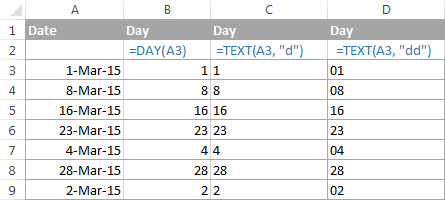## How to calculate the number of days between two dates (DAYS function)

The syntax of the Excel DAYS function is so obvious that you will hardly need any explanations :)

DAYS(end_date, start_date)

Supposing that the Start Date of your projects is in column A and the End Date in column B, you can calculate the projects' duration (i.e. the number of days between two dates) using the following formula:

`=DAYS(B2,A2)`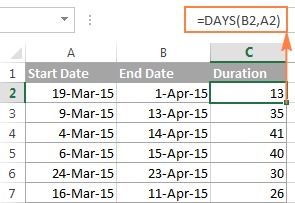This formula will work with normal dates as well as dates formatted as text. If either the start date or end date is a text value, Excel will try to convert it to date (you would use the DATEVALUE function for this). If Excel is unable to parse your text sting as a valid date, the DAYS formula will return the #VALUE! error.

## Working with days of the year in Excel

When working with days of year in Excel, you have a few functions at your disposal. Which one to choose depends on your data format and exactly what result you are after :)

#### Example 1. Get the day number of the year (1-365)

This example demonstrates how you can get the number of a certain day in a year, between 1 and 365 (1-366 in leap years) with January 1 considered day 1.

For this, you use a combination of the Excel DATE and YEAR functions:
`=A2-DATE(YEAR(A2),1,0)`

Where A2 is a cell containing the date.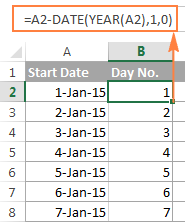And now, let's see what the formula actually does. The YEAR function retrieves the year of the date in cell A2, and passes it to the DATE(year, month, day) function, which returns the sequential number that represents a certain date.

So, in our formula, `year` is extracted from the original date (A2), `month` is 1 (January) and `day` is 0. In fact, a zero day forces Excel to return December 31 of the previous year, because we want January 1 to be treated as the 1st day. And then, you subtract the serial number returned by the DATE formula from the original date (which is also stored as a serial number in Excel) and the difference is the day of the year you are looking for. For example, January 5, 2015 is stored as 42009 and December 31, 2014 is 42004, so 42009 - 42004 = 5.

If the concept of day 0 does not seem right to you, you can use the following formula instead:

`=A2-DATE(YEAR(A2),1,1)+1`

#### Example 2. Calculate the number of days remaining in the year

To compute the number of days remaining in the year, we are going to use the DATE and YEAR functions again. The formula is based on the same approach as Example 3 above, so you are unlikely to have any difficulties with understanding its logic:

`=DATE(YEAR(A2),12,31)-A2`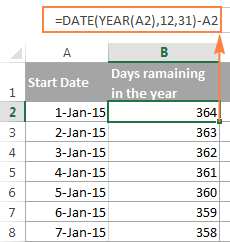If you want to know how many days remain till the end of the year based on the current date, you use the Excel TODAY() function, as follows:

`=DATE(2015,12,31)-TODAY()`

Where 2015 is the current year.

This is how you work with days of week and days of year in Excel. In the next article, we will explore Excel functions to operate on bigger time units such as weeks, months and years. Please stay tuned and thank you for reading!

### 130 Responses to "WEEKDAY, DAY and other functions to calculate days in Excel"

1.Heidi says:

If the date field is blank how do I get the return cell day of the week to stay blank? The =WEEKDAY(A2) formula is working fine and reporting as needed into the day of the week when the date is filled in, but if the date cell is blank yet (haven't added that data line yet) how do I get the day of the week cell to stay blank also?

•M says:

Try:

=iferror(weekday(a2), " ")

2.Dildar Hussain says:

Dear sir i want to account the month. from start date to end date
Example

01-12-2018 - 01-06-2018 total 06 month

3.nix says:

Say I want an "IF" formula that checks a cell (a1) with a date indicated then on a different cell (b1) it validates the indicated date on cell A1 that its already pass 3 business days once confirmed cell B1 would indicate as a "Yes" if not then a "No", is this possible?

4.James says:

Hello, I've got a problem with a specific formula.

I have 2 cells generating dates:
Todays date (using =now () ) - In the format of dd-mm-yy hh:mm:ss
Working date (using =workday(todays date,5)+time(15,0,0) so it adds +5 to working date + declares time of 15:00:00. For my purpose, I need the format to remain dd-mm-yyyy 15:00:00.

The issue that I'm having is that once the time passes 15:30:00 on today's date (=now() function) - I need the working date to increase from 5 to 6, so it becomes tomorrow's work.

Kind Regards,
James.

•James says:

I forgot to add, this will probably need to be converted to an IF function, my cell references are:

Todays Date = I2 ( =NOW() )
Working Date = I3 ( =WORKDAY(I2,5)+TIME(15,0,0) )

5.Nainan Varghese says:

Condition 1 should meet any text
Condition 2 if the date exceeds or equal the current date (eg: 16th of any month), it should return a value 2
Condition 3 if the date is between 1st and 15th of the same month, it should return a value of 1
Condition 4 if the date is of the preceding month, it should return a value of 0

My formula
=if(and(a1="A", or(today()>=date(2019,3,16), 2, if(a1="A", or(today()=date(2019,3,1),1, if(a1="A", or(today()<date(2019,3,1),0)))

This formula is not working . could you please let me know what is the syntax error

Ultimate Suite 2018.5 for Excel